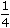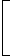# Simple Interest

### Important Formulas

1. Principal:The money borrowed or lent out for a certain period is called the principal or the sum.

2. Interest:Extra money paid for using other’s money is called interest.

3. Simple Interest (S.I.):If the interest on a sum borrowed for certain period is reckoned uniformly, then it is called simple interest.

Let Principal = P, Rate = R% per annum (p.a.) and Time = T years. Then

 (i). Simple Intereest =P x R x T100

 (ii). P =100 x S.I.; R =100 x S.I.and T =100 x S.I.. R x T P x T P x R

1

A sum of money at simple interest amounts to Rs. 815 in 3 years and to Rs. 854 in 4 years. The sum is:
 A. Rs. 650 B. Rs. 690 C. Rs. 698 D. Rs. 700

Explanation:

S.I. for 1 year = Rs. (854 – 815) = Rs. 39.

S.I. for 3 years = Rs.(39 x 3) = Rs. 117.Principal = Rs. (815 – 117) = Rs. 698.

2.

Mr. Thomas invested an amount of Rs. 13,900 divided in two different schemes A and B at the simple interest rate of 14% p.a. and 11% p.a. respectively. If the total amount of simple interest earned in 2 years be Rs. 3508, what was the amount invested in Scheme B?
 A. Rs. 6400 B. Rs. 6500 C. Rs. 7200 D. Rs. 7500 E. None of these

Explanation:

Let the sum invested in Scheme A be Rs. x and that in Scheme B be Rs. (13900 – x).

 Then,x x 14 x 2+(13900 – x) x 11 x 2= 3508 100 10028x – 22x = 350800 – (13900 x 22)6x = 45000x = 7500.

So, sum invested in Scheme B = Rs. (13900 – 7500) = Rs. 6400.

3.

A sum fetched a total simple interest of Rs. 4016.25 at the rate of 9 p.c.p.a. in 5 years. What is the sum?
 A. Rs. 4462.50 B. Rs. 8032.50 C. Rs. 8900 D. Rs. 8925 E. None of these

Explanation:

Principal

 = Rs.100 x 4016.259 x 5

 = Rs.40162545

= Rs. 8925.

4.

How much time will it take for an amount of Rs. 450 to yield Rs. 81 as interest at 4.5% per annum of simple interest?
 A. 3.5 years B. 4 years C. 4.5 years D. 5 years

Explanation:

 Time =100 x 81years = 4 years. 450 x 4.5

5.

Reena took a loan of Rs. 1200 with simple interest for as many years as the rate of interest. If she paid Rs. 432 as interest at the end of the loan period, what was the rate of interest?
 A. 3.6 B. 6 C. 18 D. Cannot be determined E. None of these

Explanation:

Let rate = R% and time = R years.

 Then,1200 x R x R= 432 10012R2 = 432R2 = 36R = 6.

6.

A sum of Rs. 12,500 amounts to Rs. 15,500 in 4 years at the rate of simple interest. What is the rate of interest?
 A. 3% B. 4% C. 5% D. 6% E. None of these

Explanation:

S.I. = Rs. (15500 – 12500) = Rs. 3000.

 Rate =100 x 3000% = 6% 12500 x 4

7.

An automobile financier claims to be lending money at simple interest, but he includes the interest every six months for calculating the principal. If he is charging an interest of 10%, the effective rate of interest becomes:
 A. 10% B. 10.25% C. 10.5% D. None of these

Explanation:

Let the sum be Rs. 100. Then,

 S.I. for first 6 months = Rs.100 x 10 x 1= Rs. 5 100 x 2

 S.I. for last 6 months = Rs.105 x 10 x 1= Rs. 5.25 100 x 2

So, amount at the end of 1 year = Rs. (100 + 5 + 5.25) = Rs. 110.25Effective rate = (110.25 – 100) = 10.25%

8.

A lent Rs. 5000 to B for 2 years and Rs. 3000 to C for 4 years on simple interest at the same rate of interest and received Rs. 2200 in all from both of them as interest. The rate of interest per annum is:
A. 5% B. 7%
C.
 7 1 % 8
D. 10%

Explanation:

Let the rate be R% p.a.

 Then,5000 x R x 2+3000 x R x 4= 2200. 100 100100R + 120R = 2200R =2200= 10. 220Rate = 10%.

9.

A sum of Rs. 725 is lent in the beginning of a year at a certain rate of interest. After 8 months, a sum of Rs. 362.50 more is lent but at the rate twice the former. At the end of the year, Rs. 33.50 is earned as interest from both the loans. What was the original rate of interest?
 A. 3.6% B. 4.5% C. 5% D. 6% E. None of these

Explanation:

Let the original rate be R%. Then, new rate = (2R)%.

Note:

Here, original rate is for 1 year(s); the new rate is for only 4 months i.e.year(s).725 x R x 1+362.50 x 2R x 1= 33.50 100 100 x 3(2175 + 725) R = 33.50 x 100 x 3(2175 + 725) R = 10050(2900)R = 10050R = 10050 = 3.46 2900Original rate = 3.46%

10.

A man took loan from a bank at the rate of 12% p.a. simple interest. After 3 years he had to pay Rs. 5400 interest only for the period. The principal amount borrowed by him was:
 A. Rs. 2000 B. Rs. 10,000 C. Rs. 15,000 D. Rs. 20,000

Explanation:

 Principal = Rs.100 x 5400= Rs. 15000. 12 x 3

11

A sum of money amounts to Rs. 9800 after 5 years and Rs. 12005 after 8 years at the same rate of simple interest. The rate of interest per annum is:
 A. 5% B. 8% C. 12% D. 15%

Explanation:

S.I. for 3 years = Rs. (12005 – 9800) = Rs. 2205.

 S.I. for 5 years = Rs.2205 x 5= Rs. 3675 3Principal = Rs. (9800 – 3675) = Rs. 6125.

 Hence, rate =100 x 3675% = 12% 6125 x 5

12.

What will be the ratio of simple interest earned by certain amount at the same rate of interest for 6 years and that for 9 years?
 A. 1 : 3 B. 1 : 4 C. 2 : 3 D. Data inadequate E. None of these

Explanation:

Let the principal be P and rate of interest be R%.Required ratio =P x R x 6100

= 6PR = 6 = 2 : 3.P x R x 9100

9PR 9

13.

A certain amount earns simple interest of Rs. 1750 after 7 years. Had the interest been 2% more, how much more interest would it have earned?
 A. Rs. 35 B. Rs. 245 C. Rs. 350 D. Cannot be determined E. None of these

Explanation:

We need to know the S.I., principal and time to find the rate.

Since the principal is not given, so data is inadequate.

14.

A person borrows Rs. 5000 for 2 years at 4% p.a. simple interest. He immediately lends it to another person at 6p.a for 2 years. Find his gain in the transaction per year.
 A. Rs. 112.50 B. Rs. 125 C. Rs. 150 D. Rs. 167.50

Explanation:

Gain in 2 years

 = Rs.5000 x 25 x 2–5000 x 4 x 24 100 100

= Rs. (625 – 400)
= Rs. 225.Gain in 1 year = Rs.225= Rs. 112.50 2